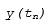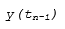## Compare Solvers

A dynamic system is simulated by computing its states at successive time steps over a specified time span, using information provided by the model. The process of computing the successive states of a system from its model is known as solving the model. No single method of solving a model suffices for all systems. Accordingly, Simulink® provides a set of programs, known as solvers, each of which embodies a particular approach to solving a model. The Configuration Parameters dialog box allows you to choose the solver best suited to your model.

### Fixed-Step Versus Variable-Step Solvers

You can choose the solvers provided by Simulink based on the way they calculate step size: fixed-step and variable-step.

Fixed-step solvers solve the model at regular time intervals from the beginning to the end of the simulation. The size of the interval is known as the step size. You can specify the step size or let the solver choose the step size. Generally, decreasing the step size increases the accuracy of the results while increasing the time required to simulate the system.

Variable-step solvers vary the step size during the simulation. They reduce the step size to increase accuracy when a model's states are changing rapidly and increase the step size to avoid taking unnecessary steps when the model's states are changing slowly. Computing the step size adds to the computational overhead at each step but can reduce the total number of steps, and hence the simulation time required to maintain a specified level of accuracy for models with rapidly changing or piecewise continuous states.

Fixed-step and variable-step solvers compute the next simulation time as the sum of the current simulation time and the step size. The Type control on the Solver configuration pane allows you to select the type of solver. With a fixed-step solver, the step size remains constant throughout the simulation. With a variable-step solver, the step size can vary from step to step, depending on the model dynamics. In particular, a variable-step solver increases or reduces the step size to meet the error tolerances that you specify.

The choice between these types depends on how you plan to deploy your model and the model dynamics. If you plan to generate code from your model and run the code on a real-time computer system, choose a fixed-step solver to simulate the model. You cannot map the variable-step size to the real-time clock.

If you do not plan to deploy your model as generated code, the choice between a variable-step and a fixed-step solver depends on the dynamics of your model. A variable-step solver might shorten the simulation time of your model significantly. A variable-step solver allows this saving because, for a given level of accuracy, the solver can dynamically adjust the step size as necessary. This approach reduces the number of steps required. The fixed-step solver must use a single step size throughout the simulation, based on the accuracy requirements. To satisfy these requirements throughout the simulation, the fixed-step solver typically requires a small step.

The `ex_multirate` example model shows how a variable-step solver can shorten simulation time for a multirate discrete model.The model generates outputs at two different rates: every 0.5 s and every 0.75 s. To capture both outputs, the fixed-step solver must take a time step every 0.25 s (the fundamental sample time for the model).

```[0.0 0.25 0.5 0.75 1.0 1.25 1.5 ...] ```

By contrast, the variable-step solver has to take a step only when the model generates an output.

```[0.0 0.5 0.75 1.0 1.5 ...] ```

This scheme significantly reduces the number of time steps required to simulate the model.

### Continuous Versus Discrete Solvers

Simulink provides both continuous and discrete solvers.

When you select a solver type, you can also select a specific solver. Both sets of solvers include discrete and continuous solvers. Discrete and continuous solvers rely on the model blocks to compute the values of any discrete states. Blocks that define discrete states are responsible for computing the values of those states at each time step. However, unlike discrete solvers, continuous solvers use numerical integration to compute the continuous states that the blocks define. When choosing a solver, determine first whether to use a discrete solver or a continuous solver.

If your model has no continuous states, then Simulink switches to either the fixed-step discrete solver or the variable-step discrete solver. If your model has only continuous states or a mix of continuous and discrete states, choose a continuous solver from the remaining solver choices based on the dynamics of your model. Otherwise, an error occurs.

Continuous solvers use numerical integration to compute a model's continuous states at the current time step based on the states at previous time steps and the state derivatives. Continuous solvers rely on the individual blocks to compute the values of the model's discrete states at each time step.

Discrete solvers exist primarily to solve purely discrete models. They compute the next simulation time step for a model and nothing else. In performing these computations, they rely on each block in the model to update its individual discrete states. They do not compute continuous states.

The solver library contains two discrete solvers: a fixed-step discrete solver and a variable-step discrete solver. The fixed-step solver by default chooses the step size and simulation rate fast enough to track state changes in the fastest block in your model. The variable-step solver adjusts the simulation step size to keep pace with the actual rate of discrete state changes in your model. This adjustment can avoid unnecessary steps and shorten simulation time for multirate models. See Sample Times in Systems for more information.

Note

You must use a continuous solver to solve a model that contains both continuous and discrete states because discrete solvers cannot handle continuous states. If, on the other hand, you select a continuous solver for a model with no states or discrete states only, Simulink software uses a discrete solver.

### Explicit Versus Implicit Continuous Solvers

You represent an explicit system by an equation

`$\stackrel{˙}{x}=f\left(x\right)$`

For any given value of x, you can compute $\stackrel{˙}{x}$ by substituting x in f(x) and evaluating the equation.

Equations of the form

`$F\left(\stackrel{˙}{x},x\right)=0$`

are considered to be implicit. For any given value of $x$, you must solve this equation to calculate $\stackrel{˙}{x}$.

A linearly implicit system can be represented by the equation

`$M\left(x\right).\stackrel{˙}{x}=f\left(x\right)$`

M(x) is called the mass matrix and $f\left(x\right)$ is the forcing function. A system becomes linearly implicit when you use physical modeling blocks in the model.

While you can apply an implicit or explicit continuous solver to solve all these systems, implicit solvers are designed specifically for solving stiff problems. Explicit solvers solve nonstiff problems. An ordinary differential equation problem is said to be stiff if the desired solution varies slowly, but there are closer solutions that vary rapidly. The numerical method must then take small time steps to solve the system. Stiffness is an efficiency issue. The more stiff a system, the longer it takes to for the explicit solver to perform a computation. A stiff system has both slowly and quickly varying continuous dynamics.

When compared to explicit solvers, implicit solvers provide greater stability for oscillatory behavior. However, implicit solvers are also computationally more expensive. They generate the Jacobian matrix and solve the set of algebraic equations at every time step using a Newton-like method. To reduce this extra cost, the implicit solvers offer a Solver Jacobian method parameter that allows you to improve the simulation performance of implicit solvers. See Choose a Jacobian Method for an Implicit Solver for more information. Implicit solvers are more efficient than explicit solvers for solving a linearly implicit system.

### One-Step Versus Multistep Continuous Solvers

The Simulink solver library provides both one-step and multistep solvers. The one-step solvers estimateusing the solution at the immediately preceding time point,, and the values of the derivative at multiple points between tn and tn-1. These points are minor steps.

Multistep solvers use the results at several preceding time steps to compute the current solution. Simulink provides one explicit multistep solver, `ode113`, and one implicit multistep solver, `ode15s`. Both are variable-step solvers.

### Single-Order Versus Variable-Order Continuous Solvers

This distinction is based on the number of orders that the solver uses to solve the system of equation. Two variable-order solvers, `ode15s` and `ode113`, are part of the solver library. They use multiple orders to solve the system of equations. Specifically, the implicit, variable-step `ode15s` solver uses first-order through fifth-order equations, while the explicit, variable-step `ode113` solver uses first-order through thirteenth-order equations. For `ode15s`, you can limit the highest order applied via the Maximum Order parameter. For more information, see Maximum Order.